# 7 Riddles for Kids to Test Your Logic

Quizzes
3 years ago

Bright Side collected some riddles that are more complicated than they may seem at first glance.

## 7.

Imagine that you're looking at this pyramid from above.

• Which of these views from above is correct?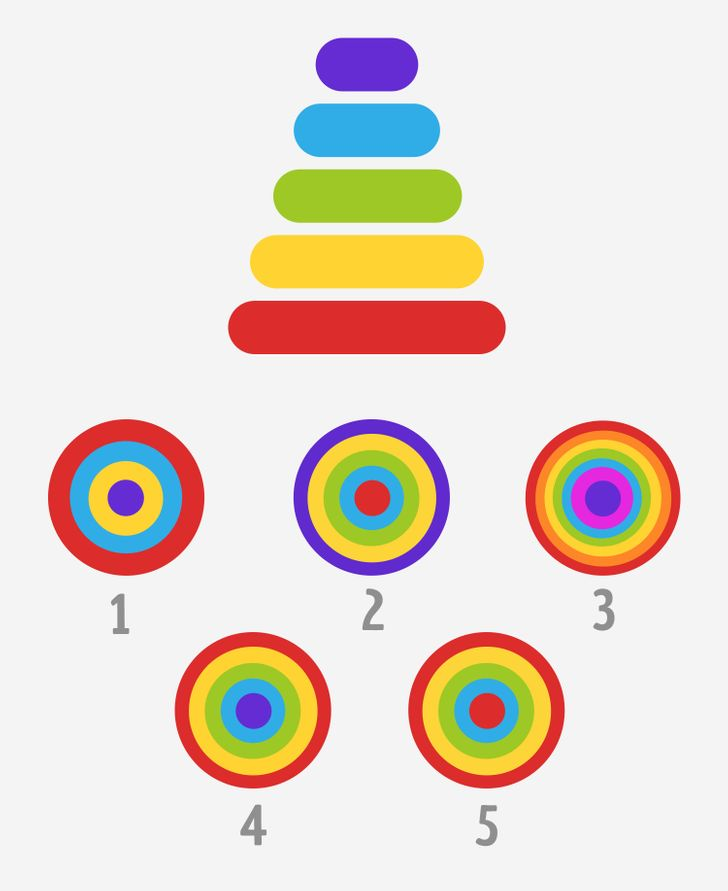## 6.

These are pieces of glass with letters. They used to make a glass board.

• Unscramble the words using the letters and find the extra word. Why is it extra?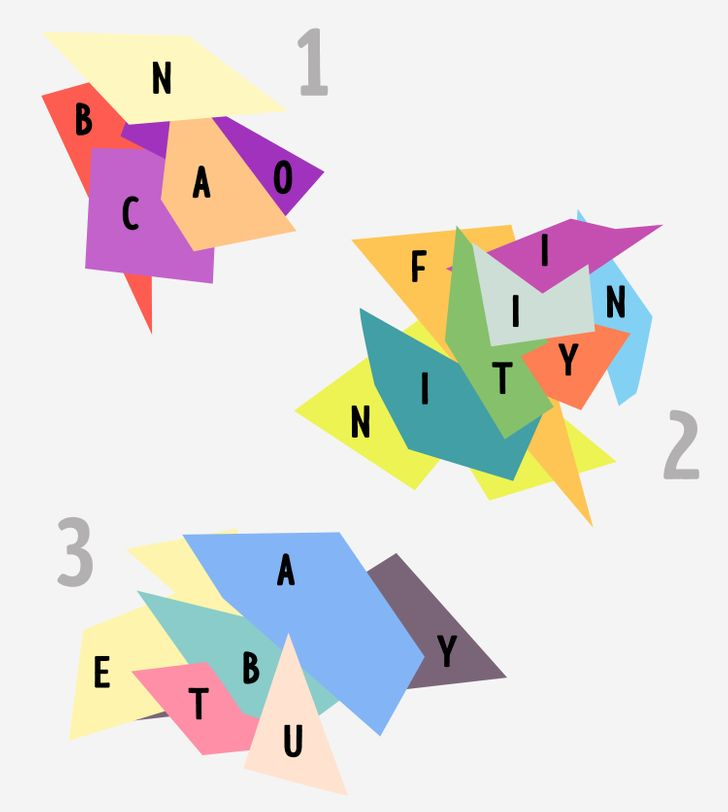## 5.

Take a look at this figure.

• Which of these answers is incorrect?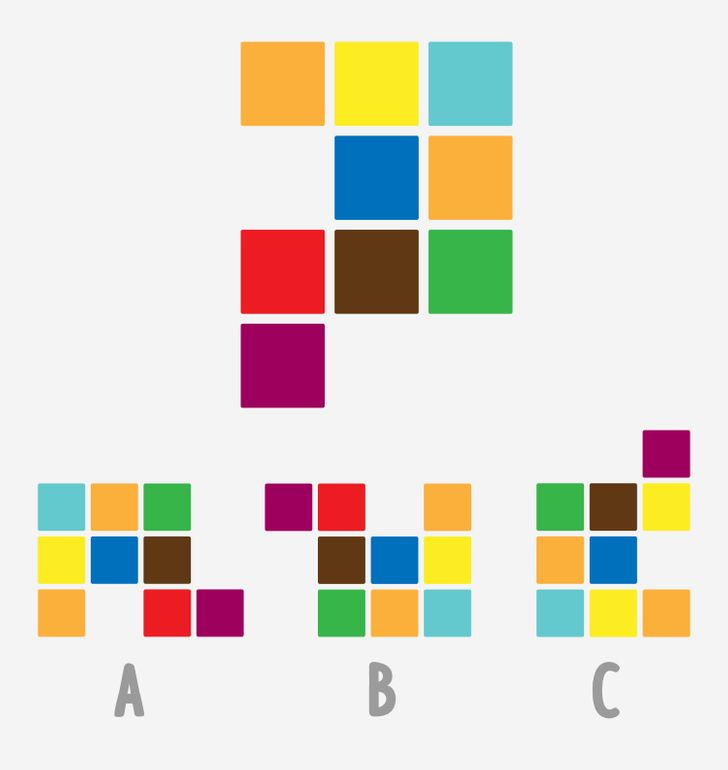## 4.

Here's a numerical series that follows certain rules.

• Which number is next? Why?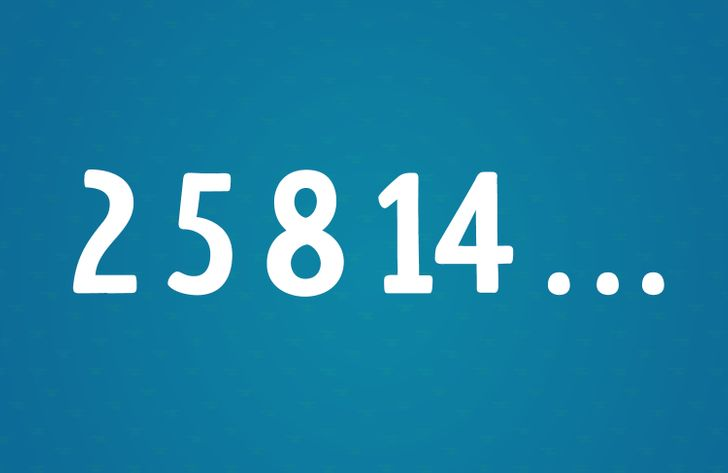## 3.

These are the surnames of famous people.

• Unscramble them and find out the extra surname.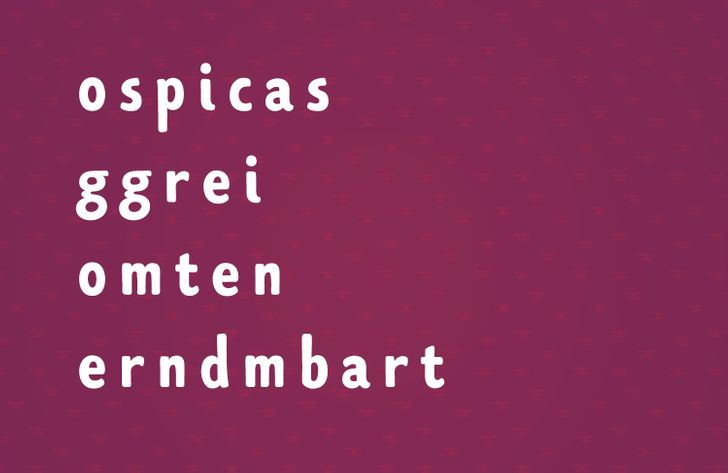## 2.

• Which number is missing? Why?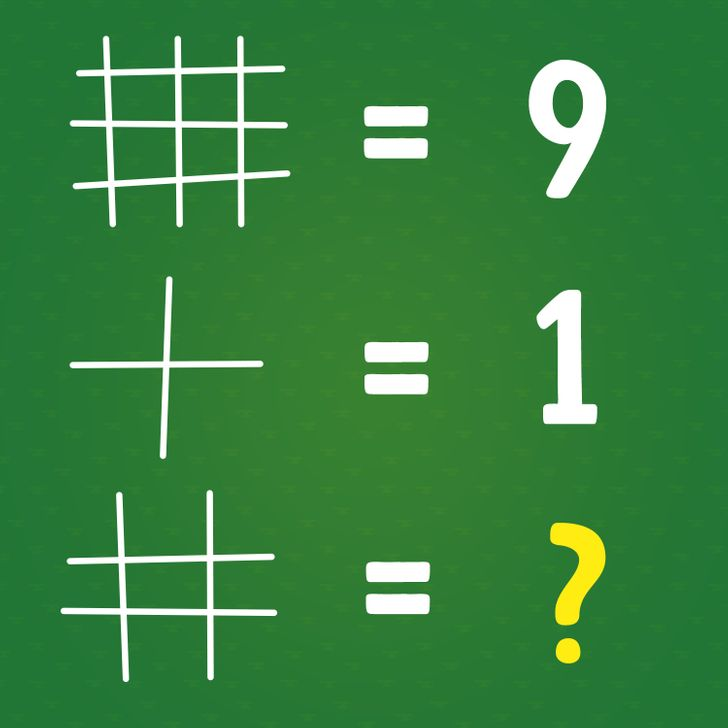## 1.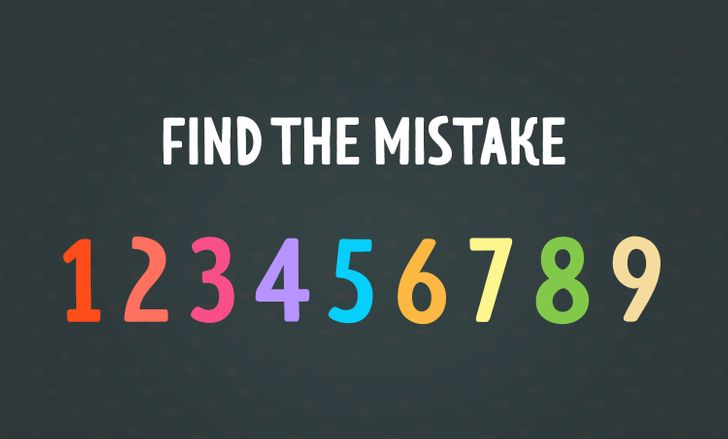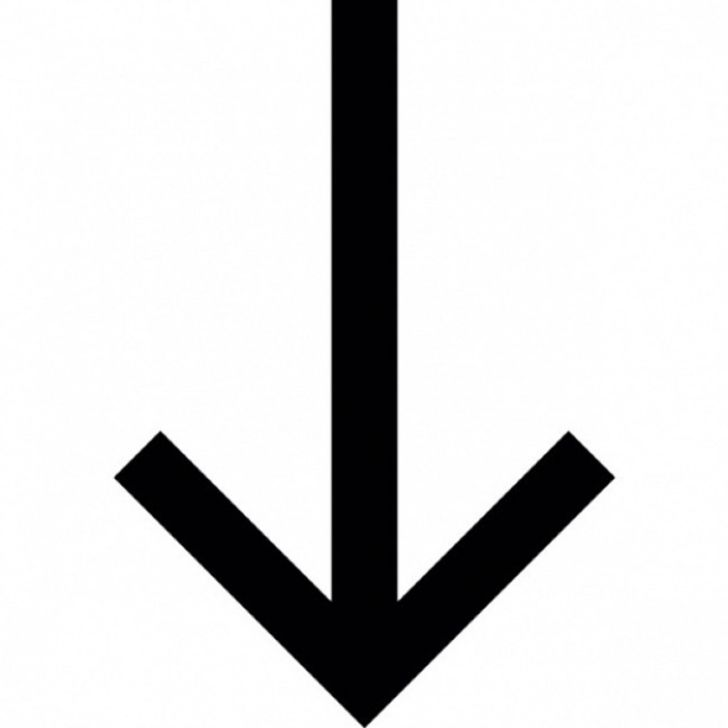## 6.

The words are "bacon," "infinity," and "beauty." The word "bacon" is extra because both "infinity" and "beauty" end in "y."

## 5.

If you turn these figures around, they all look like the given example. But the figure under letter C doesn't have the red box.

## 4.

The next number is 23. The thing here is, we increase the sum of the previous numbers by 1.

• 2 + 5 = 7; 7 + 1 = 8
• 5 + 8 = 13; 13 + 1 = 14
• 8 + 14 = 22; 22 + 1 = 23

## 3.

Here are the surnames: Picasso, Grieg, Monet, and Rembrandt. Grieg doesn't fit in this list because he's a musical composer and the rest are painters.

## 2.

The answer is the number of intersections of straight lines.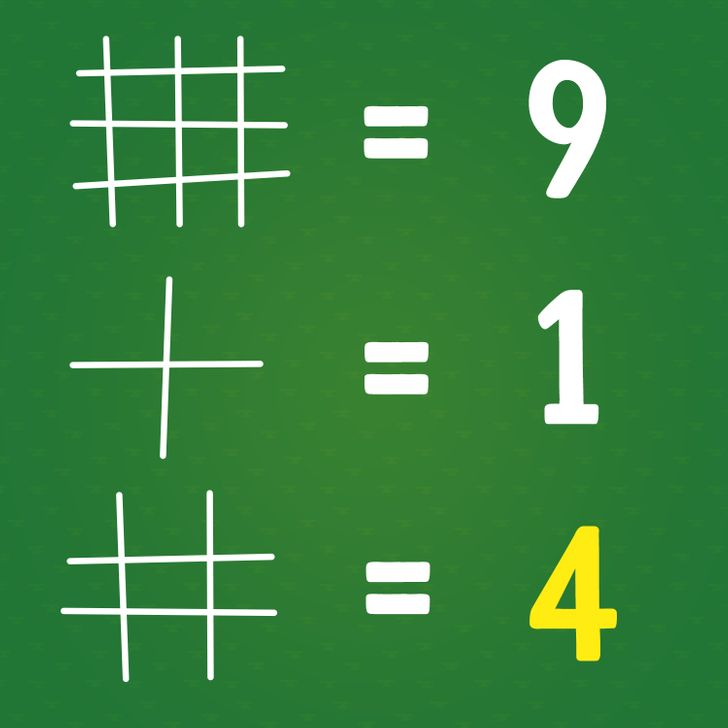## 1.

The only mistake here is the word "mistake."

Which puzzle is the most interesting? Do you know any other riddles?